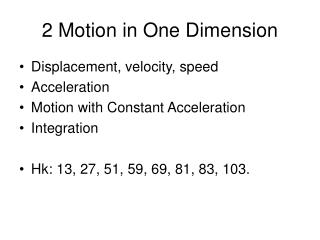Download Presentation2 Motion in One Dimension

# 2 Motion in One Dimension - PowerPoint PPT PresentationDownload Presentation## 2 Motion in One Dimension

- - - - - - - - - - - - - - - - - - - - - - - - - - - E N D - - - - - - - - - - - - - - - - - - - - - - - - - - -
##### Presentation Transcript

1. 2 Motion in One Dimension • Displacement, velocity, speed • Acceleration • Motion with Constant Acceleration • Integration • Hk: 13, 27, 51, 59, 69, 81, 83, 103.

2. Terminology • “delta” = change • “o” = initial • “ ” = final • Position • Displacement • Speed • Velocity • Acceleration

3. Concepts • Slope & derivative (Giam 12-13) • Average vs instantaneous (Giam 12-13) • Integration & area under curve (Tipler) • Acceleration/force, velocity/force • Graph shapes & equations (Giam 21)

4. Derivative Equation • Constant acceleration (constant force) • Take derivative

5. Derivative Equation

6. Integrated Equations • Integrate “a” with time

7. Integrated Equations

8. V-squared Equation • Integrate “a” over distance “x”

9. V-squared Equation

10. Average Velocity with Uniform Acceleration Uniform Acceleration = constant valued acceleration During uniform acceleration, average velocity is halfway between vo and v: 10

11. Summary • Speed = rate of travel • Velocity = speed & direction • Acceleration = rate of velocity change • Motion with Constant Acceleration • Integration

12. Problems • Describe motion (tipler 49)